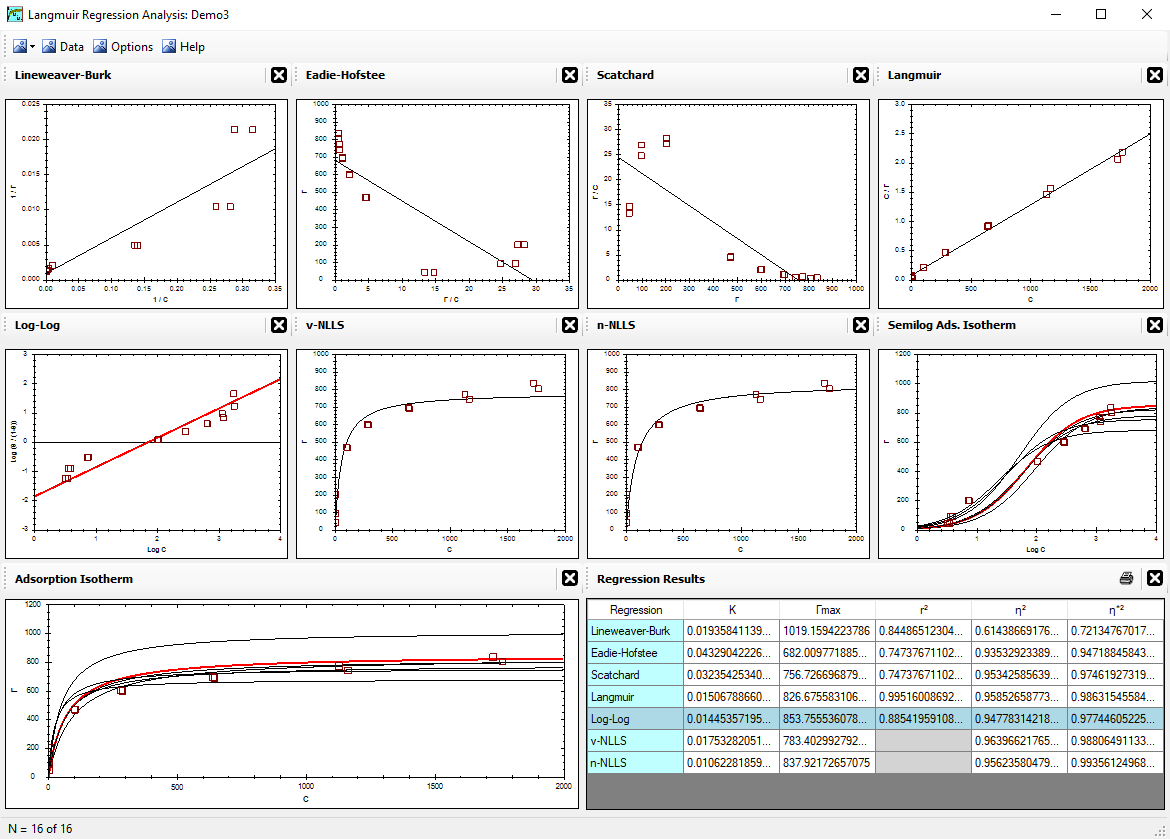Alfisol, Item 003: LMMpro, version 2.0 The Langmuir Optimization Program plus The Michaelis-Menten Optimization ProgramCorrelation Coefficient (r2) Correlation Coefficient (η2) Correlation Coefficient (η*2) Do's & Don't's References Cited

## Correlation Coefficient (η2)

The goodness-of-fit of a curve to a given set of data is based on how it compares with the goodness-of-fit of a simple horizontal line at the arithmetic average of the data. The goodness-of-fit of a curve to a set of data points is numerically expressed by the correlation coefficient (η2).

The correlation coefficient η2 coincides with r2 in the linear regression setup. The eta-square term is used here for the correlation coefficient value of the untransformed data.

The η2 assumes that a vertical minimum for the error terms corresponds to a better curve fit of the data collected. It is defined as:

 η2 =1 - Σ (yi - yp)2 Σ (yi - ya)2
where,
yp = the predicted value when x = xi,
ya = arithmetic mean value of y for all values of x, and
yi = the experimental value measured when x = xi.A perfect fit by a curve will have η2 = 1.00, whereas a poor fit by a curve will have a low value. If η2 = 0, then the predicted curve is no better than a simple average of the data collected. With all regressions, η2 < 1, always.

This is not easy to do by hand. Computers are needed to perform these calculations easily and quickly.